﻿ 1G3
 Name:    1G3

Multiple Choice
Identify the choice that best completes the statement or answers the question.

1.

Which of the following shapes shows two quarters?
 a.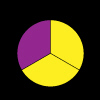c.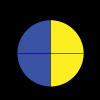b.d.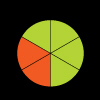2.

Which of the following does not show one whole?
 a.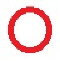c.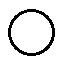b.3.

Which of the following does not show two halves?
 a.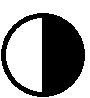c.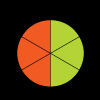b.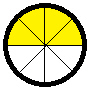d.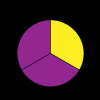4.

Which picture does not show one quarter?
 a.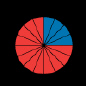c.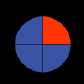b.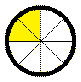d.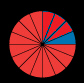5.

Which of the following shapes does not show two quarters?
 a.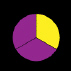c.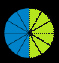b.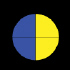6.

Which of the following pictures shows one whole?
 a.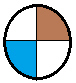c.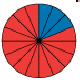b.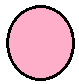d.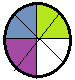7.

Which of the following shows half of a circle?
 a.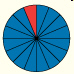c.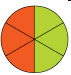b.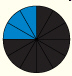d.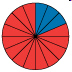8.

Which of the following shows one fourth of a circle?
 a.c.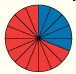b.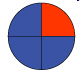d.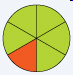9.

Which of the following pie charts shows one quarter?
 a.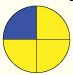c.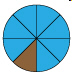b.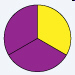d.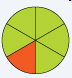10.

Which of the following does not show one fourth of a circle?
 a.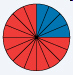c.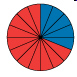b.